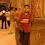## Pages

### Hashing Algorithm in C program Data Structure

This Program performs Hashing Algorithm in C. This is Part of Mumbai University MCA Colleges Data Structure C program MCA Sem 2
#include<stdio.h>
#include<conio.h>
void hash1(int,int,int *);
void hash(int,int *);
int count=1,t=0;
void main()
{

int table,key,i=0,j=0,c;
clrscr();
for(;i<=10;i++)
table[i]=0;
printf("------------------------------ HASHING ---------------------------------\n\n");
printf("Enter the no of elements you want to enter : ");
scanf("%d",&c);
for(j=0;j<c;j++)
{
printf("\n\nEnter element : ");
scanf("%d",&key);
t=0;
hash(key,table);
printf("\n\n----------------TABLE IS--------------\n\n");
for(i=0;i<=10;i++)
printf("\n\t\t%d\t%d\n",i,table[i]);
}
getch();
}

void hash(int key,int *table)
{
int h;
h=key%11;
if(*(table+h)==0)
{
*(table+h)=key;
}
else
{
t=h;
hash1(key,h,table);
}
}

void hash1(int key,int h,int *table)
{
if(count<=3)
{
if((key%7)!=0)
h=h+(key%7);
else
h=h+((key+1)%7);
h=h%11;
if(*(table+h)==0)
*(table+h)=key;
else
hash1(key,h,table);
count++;
}
else
{
h=t;
h++;
while(h!=t)
{
h=(h)%11;
if(*(table+h)==0)
{
*(table+h)=key;
printf("\n\n-----NUMBER IS INSERTED IN THE TABLE-----\n\n");
break;
}
h++;
}
if(h==t)
printf("\n\n----SPACE IS NOT AVAILABLE IN THE TABLE-----\n\n");
}
}

Hope this Program is useful to you in some sense or other. Keep on following this blog for more Mumbai University MCA College Programs. Happy Programming and Studying.

#### 1 comment:

1.Thankyou so much